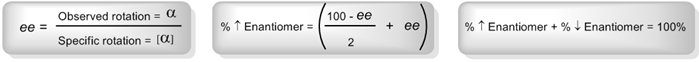Clutch Prep is now a part of Pearson
Ch. 5 - ChiralityWorksheetSee all chapters

# Calculations with Enantiomeric Percentages

See all sections
Sections
Constitutional Isomers vs. Stereoisomers
Chirality
Test 1: Plane of Symmetry
Test 2: Stereocenter Test
R and S Configuration
Enantiomers vs. Diastereomers
Atropisomers
Meso Compound
Test 3: Disubstituted Cycloalkanes
What is the Relationship Between Isomers?
Fischer Projection
R and S of Fischer Projections
Optical Activity
Enantiomeric Excess
Calculations with Enantiomeric Percentages
Non-Carbon Chiral Centers
Racemic Mixture
Achiral

Sometimes professors will ask us to solve for exact the percentage of each enantiomer in solution. For that we’ll need some new equations.Concept #1: How to solve for the percentage of each enantiomer.

Transcript

We're going to keep talking about optical activity, but it turns out that there's another way that your professor could ask these questions that's not based on the specific and observed rotations that we talked about already. It has to do with enantiomeric percentages.
Another way that your professor could ask this type of question is that they give you both the specific and the observed rotation and you have to solve for what are the percentages of each enantiomer. I know that sounds like you should be able to figure it out, and I'm sure a lot of you guys could, but it can get surprisingly tricky, so I want to go ahead and go over this now.
It turns out that there's three equations that we want to use for this. Two of them you already know. This one right here is just the shuffled around version of the equation that we were using earlier which was that observed equals specific times enantiomeric excess. That's the same equation, I just rearranged it so that we're solving for e, for enantiomeric excess now. That makes sense. The observed over the specific is your enantiomeric excess.
Then another one that you know because we've already used it is that the higher enantiomer plus the lower enantiomer always has to equal to 100%. That's just kind of common sense.
But then we've got this one in the middle that's kind of just a derivation of the definition of enantiomers. The way that it works is that if you're given the enantiomeric excess, so let's say that my enantiomeric excess is 50% and you're asked to figure out how much each enantiomer represents in that, then you would use this equation. What this equation says is that you take your percent highest enantiomer, and that would be 100 minus the ee over 2 plus the ee, and that's going to give you your percentage higher enantiomer.
Then we can use the last equation to figure out what the lower enantiomer percentage is if we know the higher one, we can easily figure out the lower one.
Let's go ahead and get started with this question. I'm going to go ahead and read it for you guys, give you guys some hints and then I'm going to have you guys solve it on your own.
It says that the specific rotation of pure S-epinephrine is positive 50. So I've been given the specific rotation that would be right here. I'm going to say it's positive 50. Calculate the ee, the enantiomeric excess, of the solution of the following observed value. The following observed value is going to be this guy right here. That means that I'm going to put here for my observed, what I'm actually getting out of it, even though I have a 50% theoretical, I'm only getting a 25%. That tells you that I have some mixture of enantiomers in here. It's definitely not 100% of the S.
Then we have to calculate the percentage of each enantiomer from the enantiomeric excess and then we have to sketch the approximate mixture in our polarimeter tube. What that means is that I want you to actually sketch out like we were doing before. Say like this part would be the S and this part would be the R or whatever, based on percentages, so if this was 50 and 50, then I would want you to draw that. These numbers are obviously wrong. I just want you to draw something like that so that you guys can kind of understand why we're only getting half of the efficacy from this 50. Instead of rotating it 50, it's only 25, why is that?
Go ahead and try to just work with the equations above. See if you can get the right answer. Fill in those three blanks below, right here. Then I'll go ahead and solve it for you guys.

Example #1: The [α] of pure S-epinephrine is +50°. Calculate the ee of a solution with an observed value of +25°. Calculate percent of each enantiomer. Then sketch the approximate mixture in our sample polarimeter tube.

Practice: The [α] of pure S-epinephrine is +50°. Calculate the ee of a solution with an observed value of -40°. Calculate percent of each enantiomer. Then sketch the approximate mixture in our sample polarimeter tube.